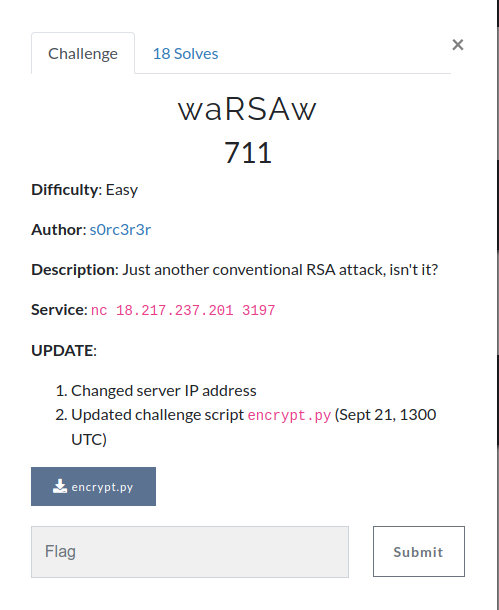# waRSAw - InCTF Internationals 2019

Intended solution of waRSAw challenge from InCTF Internationals 2019

tl;dr variant of LSB Oracle Attack on unpadded RSA

Challenge Points: 711
Challenge Solves: 18
Challenge Author: s0rc3r3r

Prerequisites:

## Challenge Description## Introduction

This variant of LSB Oracle Attacks is different as compared to the binary search LSB algorithm for recovering plaintext, in terms of time complexity and ability to recover data on session renewal.

This attacks works due to leaking of the Least Significant Bit by an unpadded RSA encryption/decryption oracle that enables the adversary to decrypt the ciphertext in len(plaintext) requests to the oracle, where len(plaintext) is the length of plaintext to be decrypted in bits.

In this article, we will try to understand the logic and details behind the variant of LSB oracle attack on unpadded RSA and hence solve the challenge waRSAw using the intended solution.

## Variant of LSB Oracle attack

Consider the following scenario:
We have access to a service that allows us to encrypt/decrypt text using unpadded RSA. The service encrypts/decrypts using it’s public key and private key respectively. But after decryption, the server only returns the last bit of the plaintext obtained. How can such a service be vulnerable?

We have seen in LSBit Oracle Attack that such a service can be exploited and the plaintext can be recovered in $log_2{N}$ requests, provided that the modulus remains the same in all the iterations.

We know that the service returns the last bit of decrypted ciphertext ie.
$(ct^d\mod n)\mod 2\equiv m\mod 2$,
where ct is the ciphertext and m is the plaintext.

How can we exploit this?

One idea is to somehow do some computation on the ciphertext to shift plaintext right by one bit in each iteration, this way we can obtain one bit of plaintext, starting from the from rightmost bit, in each iteration.

Next step is to implement this idea:
We know that any k-bit plaintext m can be written as:
$m = (a_{k-1}*2^{k-1}) + (a_{k-2}*2^{k-2}) + (a_{k-3}*2^{k-3}) + … + (a_{2}*2^{2}) + (a_{1}*2^{1}) + (a_{0}*2^{0}); \forall a_i \in { 0,1 }$

1. Get the ciphertext you want to decrypt, let us assume that it to be ct and it’s corresponding plaintext to be m
2. Send the same ciphertext to the oracle for decryption and the oracle will return the last bit of plaintext i.e. $a_0$
3. We will now craft our chosen-ciphertext attack to recover all plaintext bits

### Chosen-Ciphertext Attack

Calculate
$(ct*(2^{-e} \mod n) \mod n)^d\mod 2 \equiv m*(2^{-1}\mod n)\mod 2$

Note that the inverse of 2 is calculated over mod n and not over mod 2

From the above equation we can write:
$m*(2^{-1}\mod n)\mod 2$
$= [(a_{k-1}*2^{k-1}) + (a_{k-2}*2^{k-2}) + (a_{k-3}*2^{k-3}) + … + (a_{2}*2^{2}) + (a_{1}*2^{1}) + (a_{0}*2^{0})]*2^{-1}\mod 2$
$= [a_1 + (2^1*a_2) + … + (2^{k-2})*a_{k-1}] + (a_0*2^0)*2^{-1}$
$\equiv a_1 + (a_0*2^0)*2^{-1} \equiv y \mod 2$
$\implies y - (a_0*2^{-1})\equiv a_1\mod 2$

Now that we have the value of 2nd last bit of plaintext i.e. a1, we can adopt a similar approach to recover a2

Calculate
$(ct*(2^{-2*e} \mod n) \mod n)^d\mod 2 \equiv m*(2^{-2}\mod n)\mod 2$

From the above equation, we can write:
$m*(2^{-2}\mod n)\mod 2$
$= [(a_{k-1}*2^{k-1}) + (a_{k-2}*2^{k-2}) + (a_{k-3}*2^{k-3}) + … + (a_{2}*2^{2}) + (a_{1}*2^{1}) + (a_{0}*2^{0})]*2^{-2}\mod 2$
$= [a_2 + (2^1*a_3) + … + (2^{k-3})*a_{k-1}] + (a_1*2^1 + a_0*2^0)*2^{-2}$
$\equiv a_2 + (a_1*2^1 + a_0*2^0)*2^{-2} \equiv y \mod 2$
$\implies y - (a_1*2^1 + a_0*2^0)*2^{-2}\equiv a_2\mod 2$

We can generalise this to compute and recover ith bit of plaintext from the end

Calculate
$(ct*(2^{-i*e} \mod n) \mod n)^d\mod 2 \equiv m*(2^{-i}\mod n)\mod 2$

From the above equation, we can recover ai as:
$[(m*(2^{-i}\mod n)\mod 2) - (a_{i-1}*2^{i-1}+…+a_1*2^1 + a_0*2^0)*2^{-i}] \equiv a_i\mod 2$

What if modulus changes during plaintext recovery (Due to service reconnection etc.)? The attacker can still recover the remaining plaintext bits. He/She won’t have to start over again in case of a service restart, since some bits of plaintext would have already been covered and the next iteration to recover ai can be done directly (See the above equation).

## Solving waRSAw using variant of LSB Oracle Attack

We implement the above method to solve the challenge:

Running the above script gives us the flag: inctf{w0w_\$0_coOl_LSbit_|3r0}

In case of any query, feedback or suggestion, feel free to comment or reach me out on Twitter!